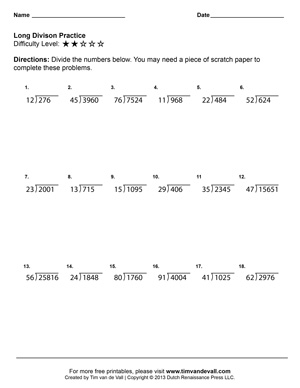# Long Division Worksheets – Printable Fourth Grade Math Worksheets

Here’s a free set of long division worksheets for fourth grade students and teachers. There are 3 long division worksheets to choose from. Each printable worksheet contains 18 math problems for students to solve and its own level of difficulty. Worksheet one contains problems with single digit divisors. Worksheet 2 contains problems with double digit divisors. Finally, worksheet 3 contains problems with triple digit divisors.

## Long Division Worksheets for Fourth Grade Math

Click on the worksheet images below to open up high resolution jpegs of the worksheets and save them to your computer.The target age group for these printable worksheets is fourth grade, but they may be suitable for third and fifth grade as well. These practice sheets are also a great way for rising fifth graders to review long division over the summer. Each of the problems should divide evenly, so the quotients should not have remainders. While there is space on the page for kids to show their work, some children may require an extra sheet of scratch paper to solve the problems.

These worksheets were created by Tim van de Vall and are copyright 2013 Dutch Renaissance Press LLC. They may be used for personal and educational purposes only.

## Useful Math Terms

Divisor: Also known as the denominator, the divisor is the number dividing the dividend. In fraction format, the divisor/denominator is the number on the bottom.

Dividend: Also known as the numerator, the dividend is the number being divided by the divisor. In fraction format, the dividend/numerator is the number on top.

Quotient: The solution to the division problem.

Dividend ÷ Divisor = Quotient

Example: 12 ÷ 3 = 4

Factor: Another term used for divisor. The divisor is a factor of the dividend.

Multiple: The product of any number and an integer. In the case of division, where the numbers divide evenly, the dividend is a multiple of the divisor.

### 2 thoughts on “Long Division Worksheets – Printable Fourth Grade Math Worksheets”

1.Desa Corridori

When I clicked the SAVE link, I get a “No Image to Save” error. When I download files, Where are they? There is no PRINT link provided, which makes a PAID subscription aggravating and actually unusable. Any suggestions?

2.